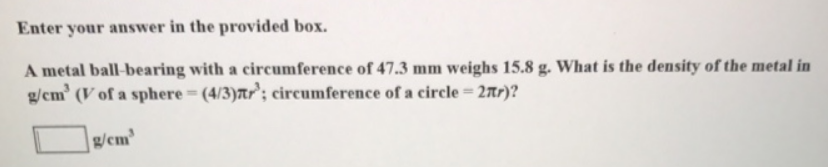# Problem: Enter your answer in the provided box.A metal ball-bearing with a circumference of 47.3 mm weighs 15.8 g. What is the density of the metal in g/cm3 (V of a sphere = (4/3)πr3; circumference of a circle = 2πr)?

###### FREE Expert Solution
100% (47 ratings)###### Problem Details

A metal ball-bearing with a circumference of 47.3 mm weighs 15.8 g. What is the density of the metal in g/cm3 (V of a sphere = (4/3)πr3; circumference of a circle = 2πr)?